# Assignment 7

Due date:Late assignments will be penalized 10% per day, and will not be accepted after.

## 1. Hand In

Each student is required to do this assignment individually and to hand in the following:
• A disk containing only two files: the source listing and executable for the program in the assignment.

• Printouts of the program and the output it produces in test data of your own choice.
Place these items in a 9"x11" envelope, with the following information clearly marked on the outside of the envelope:

Name, Student Number, Assignment Number, Course Number (T26)Assignments which are not in an envelope will not be marked

Deposit your envelope in.

### Marking Scheme

Programming standards: 10pt, Question 1: 20pt, Question 2: 40pt, Total: 70pt.

Your program must conform to the programming standards. These are described in a separate sheet.

## 2. Program Handout

In this assignment, you are given a complete implementation of the abstract type `binary tree' as well as some application code for exercising your answer to each question. Your job, in each question, is to write one application procedure that is missing.

Your procedures must be completely independent of the way binary trees are implemented - they must do all their tree manipulations using the abstract operations defined in the tree specification (the marker may use a different implementation of binary trees when she marks your program). You must use the given parts of the program without changing them.

Note that the main program writes out information about:

• max amount of memory required during this run.
• number of unreturned nodes.
• number of unreturned bytes.
The last two numbers should always be 0 (in which case they are not printed). If not, you have bugs to fix.

The file you are given (Partial7.c) contains application code for both questions. In order to select the application code relevant to the question you are working on, you must remember to define the macro `QUESTION` appropriately at the top of the file. For question 1 it should be:

`        #define QUESTION 1`
whereas for question 2 it should be:
`        #define QUESTION 2`
Also make sure you have correctly redefined `WhoAmI` to contain information by which you can be identified.

## 3. Question 1

There is a very simple, fast, algorithm for sorting an array:
• insert the elements of the array into a binary search tree

• copy the elements out of the binary search tree into the array in order. Because of the special structure of a binary search tree this step is very simple - if you traverse the tree left-to-right in `infix' order you will `visit' the elements in increasing order.
The textbook calls this TreeSort and discusses it on pp.268-269.

The program you are given does everything except step (2). You must write

`void bst_to_array (binary_tree b,element array[],int *n);`
that copies the values from binary search tree `b` into `array` in increasing order. The procedure also sets `*n`, the number of values in the array. You may assume that `*n` has been initialized to zero by the user.

## 4. Question 2

You must write the procedure `rotate_tree(t)` which `rotates' the subtree `t` according to the general algorithm described below. This procedure must be independent of the exact way trees are implemented; it must do all its tree manipulations using the abstract operations defined in the tree specification.

### Tree Rotation: The General Algorithm

Parameter `t` in `rotate_tree(t)` is the rotator node. The pivot node is the deepest node at which there is an imbalance. The rotator node is the root of the pivot's taller subtree.
`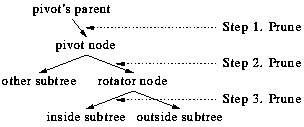`
There may be more nodes above the pivot's parent. Step 3 prunes the rotator's `inside' subtree, i.e. the one on the pivot's side. For example, if the rotator is the pivot's right subtree, then the inside subtree is the rotator's left subtree (towards the pivot rather than away from it).

Step 4: Join the pruned inside subtree to the pivot (in the place where the rotator had been).

Step 5: Join the pivot to the rotator (in the place where inside had been).

Step 6: Join the rotator to the pivot's (original) parent (in the place where the pivot had been).

Following these steps, the result of the rotation is this: the rotator has rotated up, the pivot has rotated down on the other side, and the inside subtree has jumped across to join the pivot:

`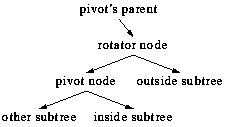`

### Example

`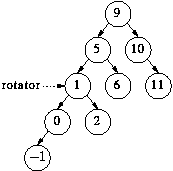`
After steps 1-3, the tree is the same but the parts that will be re-arranged have been pruned from the main tree and from one another:
`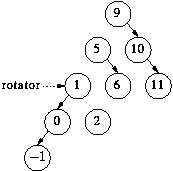`
Step 4: Join the pruned inside subtree to the pivot:
`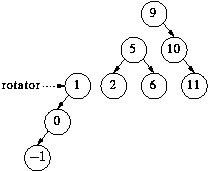`
Step 5: Join the pivot to the rotator (in the place where inside had been):
`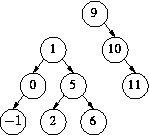`
Step 6: Join the rotator to the pivot's (original) parent:
`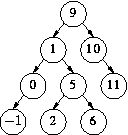`
The result of the rotation in this example is: `1' rotates up, `5' rotates down on the other side, and `2' jumps across to join `5'.

### Testing Your Code

You do not need to understand the main program in the handout in order to write your rotation procedure. The test code will repeatedly prompt you for a new value to insert in a binary tree (initially empty). After each (avl) insertion, the binary tree is printed out in the following format:
• an empty tree or non-existent subtree is represented by the character `*`.

• an non empty tree is by `[`left subtree root value right subtree`]`.
For example the tree resulting from Step 6 above would print as follows:

`[[[[* -1 *] 0 *] 1 [[* 2 *] 5 [* 6 *]]] 9 [* 10 [* 11 *]]]`

After each step, the tree should be balanced - a tree is balanced if it is empty or if the heights of its left and right subtrees are within 1. The height of a tree is the number of nodes on its longest branch - in order to do the `balancing', the program calls your `rotate_tree` procedure. If the resulting tree is not balanced, the program will let you know, and that means there is a bug in your `rotate_tree` procedure. The printed version of the tree may (or may not) give you a clue.